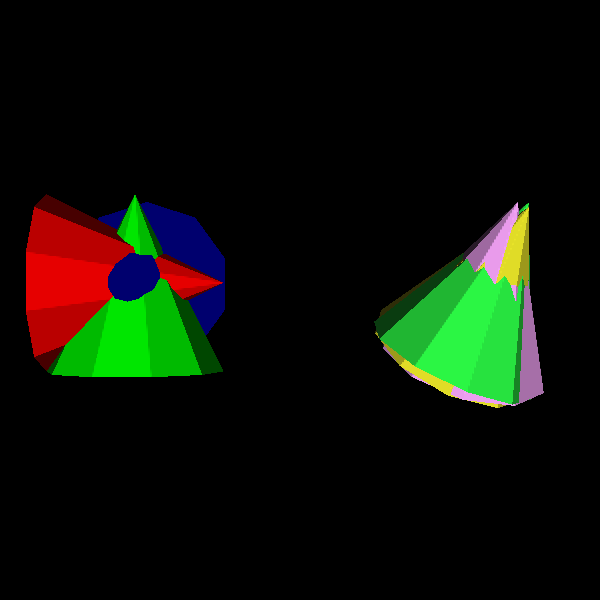# Fury Cone Actor#

This example shows how to use the cone actor.

```import numpy as np
from fury import window, actor
```

First thing, you have to specify centers, directions, and colors of the cone

```centers = np.zeros([3, 3])
dirs = np.identity(3)
colors = np.identity(3)
```

The below cone actor is generated by repeating the cone primitive.

```cone_actor1 = actor.cone(centers, dirs, colors=colors, heights=1.5)
```

repeating what we did but this time with random directions, and colors Here, we’re using vtkConeSource to generate the cone actor

```cen2 = np.add(centers, np.array([3, 0, 0]))
dir2 = np.random.rand(5, 3)
cols2 = np.random.rand(5, 3)

cone_actor2 = actor.cone(cen2, dir2, colors=cols2,
heights=1.5, use_primitive=False)

scene = window.Scene()
```

Adding our cone actors to scene.

```scene.add(cone_actor1)